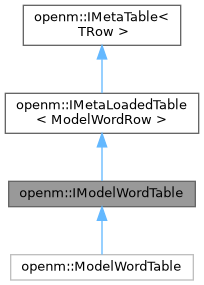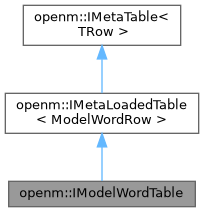OpenM++ runtime library (libopenm)
openm::IModelWordTable Struct Referenceabstract

model_word table public interface. More...

Inheritance diagram for openm::IModelWordTable:[legend]
Collaboration diagram for openm::IModelWordTable:[legend]

## Public Member Functions

virtual const ModelWordRowbyKey (int i_modelId, int i_langId, const string &i_code) const =0
binary search row by primary key: model id, language and code, return NULL if not found.

virtual IRowBaseVecrowsRef (void)=0
get reference to list of all table rows.Public Member Functions inherited from openm::IMetaLoadedTable< ModelWordRow >
virtual const IRowBaseVecrowsCRef (void) const=0
get const reference to list of all table rows.

IRowBaseVec::difference_type rowCount (void) const
return number of rows.

vector< ModelWordRowrows (void) const
get list of loaded table rows.

const ModelWordRowfirstRow (void) const
return first table row or NULL if table is empty.

vector< ModelWordRowfindAll (RowEqual i_cmp) const
get list of rows by predicate: all rows where comparator to i_row return true.

const ModelWordRowfindFirst (RowEqual i_cmp) const
get first row by predicate or NULL if not found: first row where comparator to i_row return true.

const ModelWordRowbyIndex (IRowBaseVec::difference_type i_index) const
return row value by index or NULL if out of range.

IRowBaseVec::difference_type indexOf (RowEqual i_cmp, IRowBaseVec::difference_type i_startPos=0) const
get first row by predicate or -1 if not found: first row where comparator to i_row return true.

IRowBaseVec::size_type countOf (RowEqual i_cmp) const
count rows by predicate: where comparator to i_row return true.

## Static Public Member Functions

static IModelWordTablecreate (IDbExec *i_dbExec, int i_modelId=0, int i_langId=-1)
create new table object and load table rows sorted by primary key: model id, language id, word code. More...

static IModelWordTablecreate (IRowBaseVec &io_rowVec)
create new table rows by swap with supplied vector of rows.Public Types inherited from openm::IMetaLoadedTable< ModelWordRow >
typedef function< bool(const ModelWordRow &i_dbRow)> RowEqual
db table row comparator.Protected Member Functions inherited from openm::IMetaLoadedTable< ModelWordRow >
const ModelWordRowfindKey (const IRowBaseUptr &i_row) constStatic Protected Member Functions inherited from openm::IMetaLoadedTable< ModelWordRow >
load table: return vector of selected rows sorted by primary key.Static Protected Member Functions inherited from openm::IMetaTable< TRow >
static vector< TRow > rows (const IRowBaseVec &i_rowVec)
get list of table rows.

## Detailed Description

model_word table public interface.

## ◆ create()

 IModelWordTable * IModelWordTable::create ( IDbExec * i_dbExec, int i_modelId = `0`, int i_langId = `-1` )
static

create new table object and load table rows sorted by primary key: model id, language id, word code.

if i_modelId > 0 then select only rows where model_id = i_modelId if i_langId >= 0 then select only rows where lang_id = i_langId

The documentation for this struct was generated from the following files: